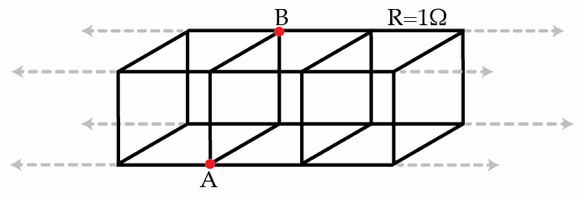# Long transmission line

Certain transmission lines can be modeled as a long 3D circuit as shown in the figure below. Here, all the the wires have equal length and electrical resistance $R=1~\Omega$. Determine the equivalent resistance in Ohms between the points A and B. Assume the transmission line to be infinite.×

Problem Loading...

Note Loading...

Set Loading...